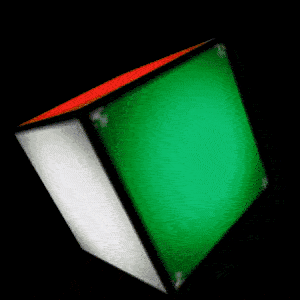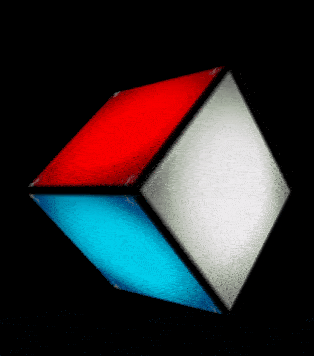# OpenGL 学习系列---观察矩阵

##### 标签: OpenGLCamera

OpenGL 投影矩阵 这篇文章中，讲述了 OpenGL 坐标系统中的投影矩阵，有两种类型的投影矩阵，分别是正交投影和透视投影。$$V_{clip}=M_{projection} \cdot M_{view} \cdot M_{model} \cdot V_{local}$$

## 确定摄像机位置## Matrix.lookAt 函数

	 /**
* Defines a viewing transformation in terms of an eye point, a center of
* view, and an up vector.
*
* @param rm returns the result
* @param rmOffset index into rm where the result matrix starts
* @param eyeX eye point X
* @param eyeY eye point Y
* @param eyeZ eye point Z
* @param centerX center of view X
* @param centerY center of view Y
* @param centerZ center of view Z
* @param upX up vector X
* @param upY up vector Y
* @param upZ up vector Z
*/
public static void setLookAtM(float[] rm, int rmOffset,
float eyeX, float eyeY, float eyeZ,
float centerX, float centerY, float centerZ, float upX, float upY,
float upZ)


## 移动相机观察内容

### 旋转移动相机var num = 0
var RotateNum  = 360 // 绕 Y 轴做圆形旋转，把圆分成 360 份
val radian = (2 * Math.PI / RotateNum).toFloat() // 每一份对应的弧度
override fun onSurfaceChanged(gl: GL10?, width: Int, height: Int) {
//... 省略代码
// 通过 RxJava 的 interval 操作符，每隔 30 毫秒，移动相机观察角度
Observable.interval(30, TimeUnit.MILLISECONDS)
.subscribe {
eyeX = eyeDistance * Math.sin((radian * num).toDouble()).toFloat()
eyeZ = eyeDistance * Math.cos((radian * num).toDouble()).toFloat()
num++
if (num > 360) {
num = 0
}
}
// 设置观察矩阵
MatrixState.setCamera(eyeX, eyeY, eyeZ, lookX, lookY, lookZ, upX, upY, upZ)
// 让物体稍微向 Z 轴负方向倾斜一定角度
MatrixState.rotate(-30f, 0f, 0f, 1f)
}


    override fun onDrawFrame(gl: GL10?) {
//... 省略代码
MatrixState.setCamera(eyeX, eyeY, eyeZ, lookX, lookY, lookZ, upX, upY, upZ)
GLES20.glUniformMatrix4fv(uViewMatrixAttr, 1, false, MatrixState.getVMatrix(), 0)
//... 省略代码
}


### 前后移动相机override fun onSurfaceChanged(gl: GL10?, width: Int, height: Int) {
super.onSurfaceChanged(gl, width, height)
var isPlus = true
var distance = eyeZ
Observable.interval(100, TimeUnit.MILLISECONDS)
.subscribe {
distance = if (isPlus) distance + 0.1f else distance - 0.1f
if (distance < 2.0f) {
isPlus = true
}
if (distance > 5.0f) {
isPlus = false
}
eyeZ = distance
updateCamera()
}
// 将物体调整一下，可以看到三个面
MatrixState.rotate(-45f, 0f, 1f, 0f)
MatrixState.rotate(45f, 1f, 0f, 0f)
}


## 小结

https://github.com/glumes/AndroidOpenGLTutorial

「真诚赞赏，手留余香」#### 欢迎扫码关注微信公众号：【音视频开发进阶】，获得最新文章推送~~~